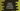# Python Tutorial : Part 3 : Python Datatype### What is DataType in python :

Each variable stored in memory has a datatype. Datatypes are actually classes and if you create any variable of a specific datatype, it is an object. Python’s standard datatypes can be grouped into mainly four different classes : 1. Numeric 2. Sequences 3. Sets 4. Mapping

### 1. Numeric :

Numeric type includes : a) Integer (int): In python, the value of an integer can be of unlimited length. ( it only depends on the available memory ) b)Long (long): Long integers of unlimited length. But exists only in python 2.x. c)Float (float): Floating point numbers. Integers and floating point numbers are separated by a decimal point. The maximum no. of places after the decimal for a float is 15 in python. d)Complex numbers (complex): Complex numbers are represented as ( x + yj ) where x is the real part and y is the imaginary part.

Integer, long, float and complex all are immutable types.

We can use type() function to check which class a variable belongs to.

``````count1 = 15
print(“count1 type ", type(count1))

count2 = 15.5
print(“count2 type ", type(count2))

count3 = 15 + 6j
print(“count3 type ", type(count3))
``````

Run the above program and you can find the datatype for each variable.

### 2. Sequences :

Sequences type includes :

### a) Python String (str) :

The string is actually a sequence of 8-bit characters ( in Python 2.x ) or a sequence of Unicode characters ( in Python 3.x ). Strings are immutable i.e. we cannot change any character of a String. To represent a String in python, single quote or double quote is used. For multiline strings, a triple quote is used. To print a particular character inside a String, use stringName[position]. Similarly, to print characters in a range, use stringName[firstPosition,lastPosition + 1].

Try to run the following program and check the results :

``````str1 = 'This is a String'
print str1

str2 = "This is also a String"
print str2

str3 = ''' This is a multiline
String '''
print str3

str4 = """ This is also a multiline
String """
print str4

print("Printing the first character of str1 "+str1)
print("Printing the first word of str1 "+str1[0:4])``````

### b) Python list :

Lists are ordered sequence of variables. The list is mutable, i.e. you can alter any item of a list. Also, list can contain items of different types. To declare a list bracket [.] is used. If you want to print or alter a variable inside a list, you can use listname[variablePosition]. Check the following example :

``````myList = [1,"one",1.0]
print myList

print ("first element of the list is "+str(myList))

myList = "2"

print ("first element is changed")

print ("now , first element is "+myList)

At first, the first element was 1. We have changed it to “2”.``````

### c) Python Tuple :

Python tuple is same as a list, the only difference is that it is immutable. To represent tuple in python, we use parentheses (). Let’s try with the above example for a tuple :

``````myList = (1,"one",1.0)
print myList

print ("first element of the list is "+str(myList))

myList = "2"

print ("first element is changed")

print ("now , first element is "+myList)
``````

It will throw an error on myList = “2” line as we cannot change any values in the tuple.

### 3) Python Sets :

Sets type includes :

### a) Set :

Set is an unordered collection of unique objects. Each item is separated by a comma inside braces {}. We can also pass a list to the set function to create a new set.

Check the below example :

``````mySet1 = set("python")
print mySet1

myList = (1,"one","two")
mySet2 = set(myList)
print mySet2

mySet3 = set([1,1,1,1,1,1])
print mySet3
``````

The output will be like below :

``````set(['h', 'o', 'n', 'p', 't', 'y'])
set([1, 'two', 'one'])
set()
``````

You can see that mySet3 contains only one “1” as all values of a set should be unique.

We can perform set operations like add, remove, intersection, union etc on sets.

### b) Frozen Sets :

The frozen set is similar as sets but they are immutable.

### Python Dictionary:

Python Dictionary is a collection of key-value pairs. Dictionary is an unordered collection. To get any value from the dictionary, we must know the key. Dictionaries are defined within braces {}. Each element or key-value pairs can be of any type.

Check the example below :

``````myDictionary = {"key1":'value1',"key2":2}

print ("Element for key key2 "+str(myDictionary["key2"]))
``````

It will print 2 as value for key “key2” is 2.